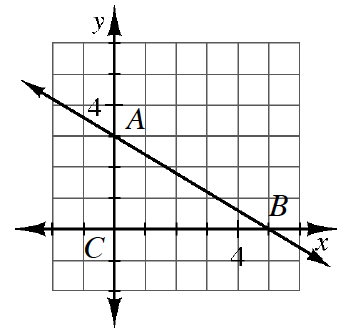### Home > INT2 > Chapter 5 > Lesson 5.2.5 > Problem5-115

5-115.Examine the graph of line $\overleftrightarrow { A B }$ at right.

1. Write the equation of $\overleftrightarrow { A B }$.

Identify the slope and $y$-intercept from the graph and use the equation $y = mx + b$ to write the equation of the line.

2. What are the $x$- and $y$-intercepts of the line?

3. Calculate the area and perimeter of $ΔABC$.

The area of a triangle is $(0.5)(\text{height)(base)}$.
Find the length of the hypotenuse by using the given leg lengths and the Pythagorean Theorem.

4. Write an equation of the line through $A$ that is perpendicular to $\overleftrightarrow { A B }$.

The slope of a perpendicular line is negative the reciprocal of the original line.